# Random variables, transformations of

(diff) ← Older revision | Latest revision (diff) | Newer revision → (diff)

The determination of functions of given arbitrary random variables for which the probability distributions possess given properties.

Example 1. Letbe a random variable having a continuous and strictly increasing distribution function. Then the random variablehas a uniform distribution on the interval, and the random variable(whereis the standard normal distribution function) has a normal distribution with parameters 0 and 1. Conversely, the formula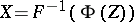enables one to obtain a random variablethat has the given distribution functionfrom a random variable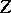with a standard normal distribution.

Transformations of random variables are often used in connection with limit theorems of probability theory. For example, let a sequence of random variablesbe asymptotically normal with parameters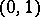. One then poses the problem of constructing simple (and simply invertible) functionssuch that the random variables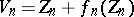are "more normal" than.

Example 2. Let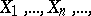be independent random variables, each having a uniform distribution on, and put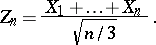By the central limit theorem,If one sets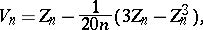then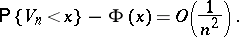Example 3. The random variables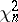,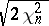andare asymptotically normal as(see "Chi-squared" distribution). The uniform deviation of the corresponding distribution functions from their normal approximations becomes less than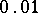forwhen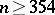, and for(the Fisher transformation) — when; for(the Wilson–Hilferty transformation) whenthis deviation does not exceed.

Transformations of random variables have long been applied in problems of mathematical statistics as the basis for constructing simple asymptotic formulas of high precision. Transformations of random variables are also used in the theory of stochastic processes (for example, the method of the "single probability space" ).

How to Cite This Entry:
Random variables, transformations of. Encyclopedia of Mathematics. URL: http://encyclopediaofmath.org/index.php?title=Random_variables,_transformations_of&oldid=28561
This article was adapted from an original article by V.I. PagurovaYu.V. Prokhorov (originator), which appeared in Encyclopedia of Mathematics - ISBN 1402006098. See original article